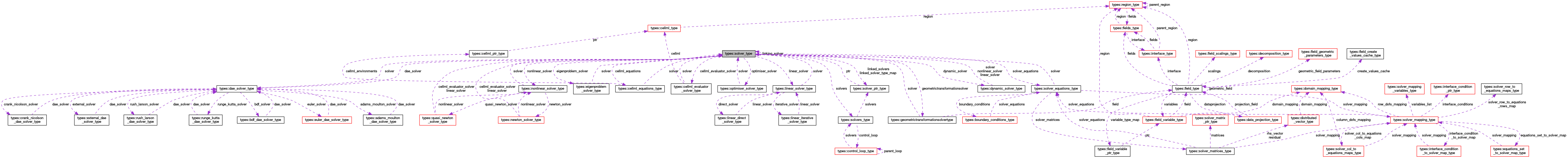OpenCMISS-Iron Internal API Documentation
types::solver_type Type Reference

Contains information on the type of solver to be used. More...

Collaboration diagram for types::solver_type:[legend]

## Public Attributes

type(solvers_type), pointer solvers
A pointer to the control loop solvers. Note that if this is a linked solver this will be NULL and solvers should be accessed through the linking solver. More...

integer(intg) global_number
The global number of the solver in the list of solvers. More...

A pointer to any solver that is linking to this solver. More...

The number of linked solvers. More...

LINKED_SOLVER_TYPE_MAP(linked_solver_idx). The map from the available linked solver types to the linked solver types that are defined for the solver. linked_solver_idx varies from 1 to SOLVER_ROUTINES::SOLVER_NUMBER_OF_SOLVER_TYPES. If the particular linked solver type has not been defined on the solver then the LINKED_SOLVER_TYPE_MAP will be NULL. More...

logical solver_finished
Is .TRUE. if the solver has finished being created, .FALSE. if not. More...

type(varying_string) label
A user defined label for the solver. More...

integer(intg) output_type
The type of output required. More...

integer(intg) solve_type
The type of the solver. More...

type(linear_solver_type), pointer linear_solver
A pointer to the linear solver information. More...

type(nonlinear_solver_type), pointer nonlinear_solver
A pointer to the nonlinear solver information. More...

type(dynamic_solver_type), pointer dynamic_solver
A pointer to the dynamic solver information. More...

type(dae_solver_type), pointer dae_solver
A pointer to the differential-algebraic equation solver information. More...

type(eigenproblem_solver_type), pointer eigenproblem_solver
A pointer to the eigenproblem solver information. More...

type(optimiser_solver_type), pointer optimiser_solver
A pointer to the optimiser solver information. More...

type(cellml_evaluator_solver_type), pointer cellml_evaluator_solver
A pointer to the CellML solver information. More...

type(geometrictransformationsolvertype), pointer geometrictransformationsolver
A pointer to the geometric transformation solver information. More...

type(solver_equations_type), pointer solver_equations
A pointer to the solver equations. More...

type(cellml_equations_type), pointer cellml_equations
A pointer to the CellML equations. More...

## Detailed Description

Contains information on the type of solver to be used.

OPENCMISS::CMISSSolverType

Definition at line 2777 of file types.f90.

## Member Data Documentation

 type(cellml_equations_type), pointer types::solver_type::cellml_equations

A pointer to the CellML equations.

Definition at line 2800 of file types.f90.

 type(cellml_evaluator_solver_type), pointer types::solver_type::cellml_evaluator_solver

A pointer to the CellML solver information.

Definition at line 2797 of file types.f90.

 type(dae_solver_type), pointer types::solver_type::dae_solver

A pointer to the differential-algebraic equation solver information.

Definition at line 2794 of file types.f90.

 type(dynamic_solver_type), pointer types::solver_type::dynamic_solver

A pointer to the dynamic solver information.

Definition at line 2793 of file types.f90.

 type(eigenproblem_solver_type), pointer types::solver_type::eigenproblem_solver

A pointer to the eigenproblem solver information.

Definition at line 2795 of file types.f90.

 type(geometrictransformationsolvertype), pointer types::solver_type::geometrictransformationsolver

A pointer to the geometric transformation solver information.

Definition at line 2798 of file types.f90.

 integer(intg) types::solver_type::global_number

The global number of the solver in the list of solvers.

Definition at line 2779 of file types.f90.

 type(varying_string) types::solver_type::label

A user defined label for the solver.

Definition at line 2785 of file types.f90.

 type(linear_solver_type), pointer types::solver_type::linear_solver

A pointer to the linear solver information.

Definition at line 2791 of file types.f90.

LINKED_SOLVER_TYPE_MAP(linked_solver_idx). The map from the available linked solver types to the linked solver types that are defined for the solver. linked_solver_idx varies from 1 to SOLVER_ROUTINES::SOLVER_NUMBER_OF_SOLVER_TYPES. If the particular linked solver type has not been defined on the solver then the LINKED_SOLVER_TYPE_MAP will be NULL.

SOLVER_ROUTINES::SolverTypes

Definition at line 2783 of file types.f90.

Definition at line 2782 of file types.f90.

A pointer to any solver that is linking to this solver.

Definition at line 2780 of file types.f90.

 type(nonlinear_solver_type), pointer types::solver_type::nonlinear_solver

A pointer to the nonlinear solver information.

Definition at line 2792 of file types.f90.

Definition at line 2781 of file types.f90.

 type(optimiser_solver_type), pointer types::solver_type::optimiser_solver

A pointer to the optimiser solver information.

Definition at line 2796 of file types.f90.

 integer(intg) types::solver_type::output_type

The type of output required.

SOLVER_ROUTINES::OutputTypes,SOLVER_ROUTINES

Definition at line 2787 of file types.f90.

 integer(intg) types::solver_type::solve_type

The type of the solver.

SOLVER_ROUTINES::SolverTypes,SOLVER_ROUTINES

Definition at line 2789 of file types.f90.

 type(solver_equations_type), pointer types::solver_type::solver_equations

A pointer to the solver equations.

Definition at line 2799 of file types.f90.

 logical types::solver_type::solver_finished

Is .TRUE. if the solver has finished being created, .FALSE. if not.

Definition at line 2784 of file types.f90.

 type(solvers_type), pointer types::solver_type::solvers

A pointer to the control loop solvers. Note that if this is a linked solver this will be NULL and solvers should be accessed through the linking solver.

Definition at line 2778 of file types.f90.